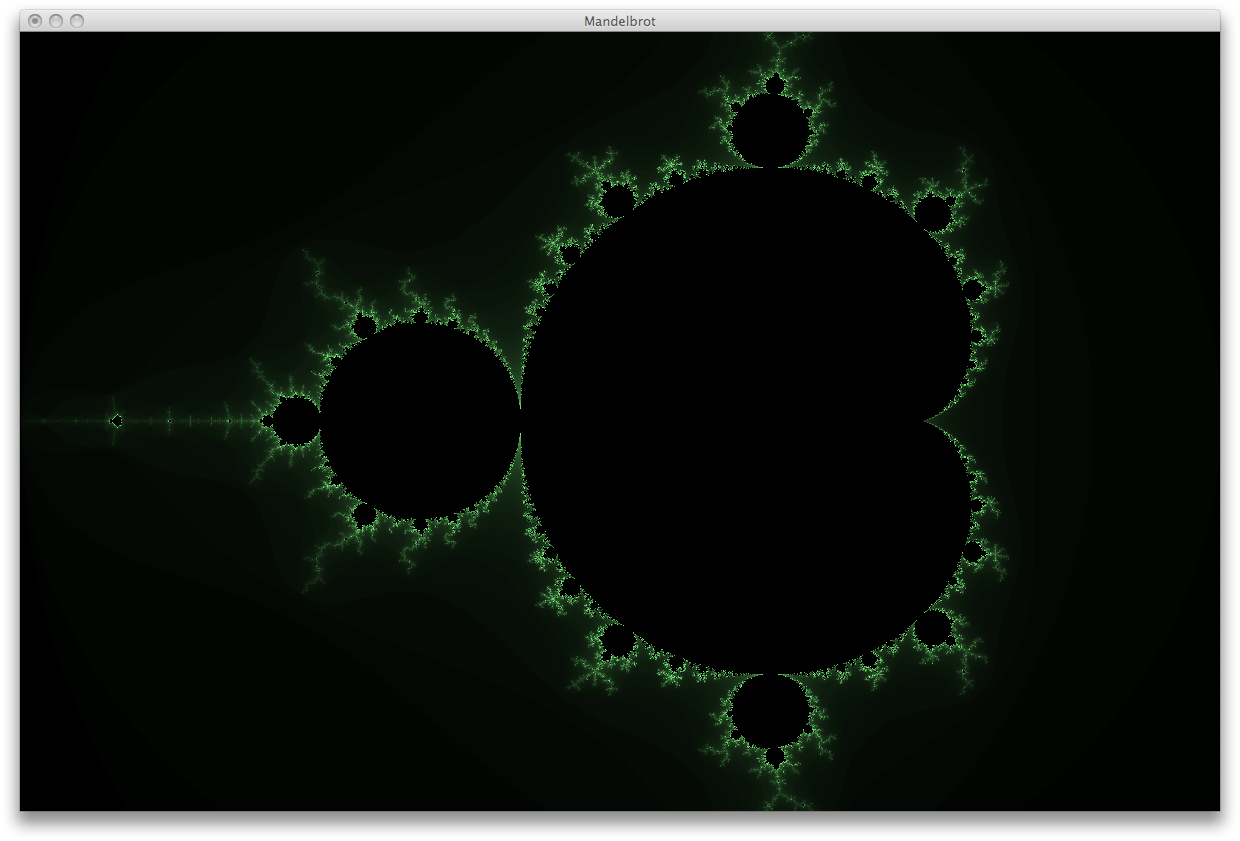# Dan McKinleyMath, Programming, and Minority Reports

#### Haskell Mandelbrot Set December 25th, 2007

Some people sing carols every XXX-mas, I get bored and write the Mandelbrot Set program in whatever my favorite language happens to be that year. I thought the brevity of the output this year (Haskell) was kinda neat.

``````import Graphics.UI.GLUT
import Data.Int
import Data.Complex

iterations = 400

x // y = fromIntegral x / fromIntegral y

-- Divides [a] into [[a], [a], ...] with each sublist of length n,
-- except the last sublist which has length <= n.
chunkify n [] = []
chunkify n xs = let (xs', rest) = splitAt n xs
in xs' : chunkify n rest

-- Converts a coordinate in screen space to a vertex.
pix2vert (Size w h) (x, y) = Vertex2 ((3 // w * fromIntegral x) - 2.0)
((2 // h * fromIntegral y) - 1.0)

-- List of all of the vertices that represent screen pixels.
vertices :: IO [Vertex2 GLfloat]
vertices = get windowSize >>= \(Size w h) ->
return \$ [pix2vert (Size w h) (x, y) | x <- [0..w-1], y <- [0..h-1]]

-- Gets the color for a number of iterations.
color3 r g b = Color3 r g b
getcolor :: Int -> Color3 Float
getcolor iter | iter == iterations = color3 0 0 0
| otherwise          = color3 (amt*0.5) amt (amt*0.5)
where amt = iter // iterations

-- Returns the number of iterations <= the maximum iterations of the
-- Mandelbrot set at the given vertex.
mandel (Vertex2 r i) = length . takeWhile (\z -> magnitude z <= 2) .
take iterations \$ iterate (\z -> z^2 + (r :+ i)) 0

-- plots one point.
drawVert v = do color . getcolor \$ mandel v
vertex v

-- draws all the vertices in slices (to update the display while drawing).
display' chunks = do mapM_ (\vs -> do renderPrimitive Points \$ mapM_ drawVert vs
flush) chunks
displayCallback \$= display

-- draws the whole fractal
display = do clear [ ColorBuffer ]
displayCallback \$= (vertices >>= display' . chunkify 256)
get currentWindow >>= postRedisplay

main = do
getArgsAndInitialize
initialDisplayMode \$= [ SingleBuffered, RGBMode ]
initialWindowSize \$= Size 1200 1024
initialWindowPosition \$= Position 100 100
createWindow "Mandelbrot"
clearColor \$= Color4 0 0 0 0
matrixMode \$= Projection